# Geometry of Congruent & Similar Figures Chapter Exam

Exam Instructions:

Choose your answers to the questions and click 'Next' to see the next set of questions. You can skip questions if you would like and come back to them later with the yellow "Go To First Skipped Question" button. When you have completed the practice exam, a green submit button will appear. Click it to see your results. Good luck!

### Page 1

#### Question 3 3. If the two stars are similar, what is the ratio of all of the corresponding sides (smaller to larger)?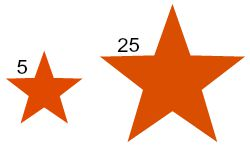#### Question 4 4. Are the two triangles similar? If so, what is the ratio of their corresponding sides?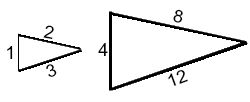### Page 2

#### Question 6 6. The two triangles are similar. The perimeter of Triangle A is 15 meters. What is the perimeter of Triangle B?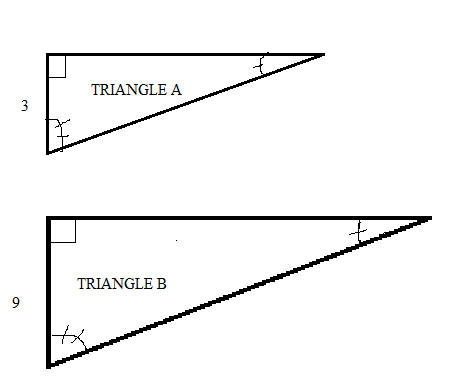#### Question 7 7. Triangle A and Triangle B are similar. The red lines represent the altitude of each triangle. What is the ratio of CD to EF?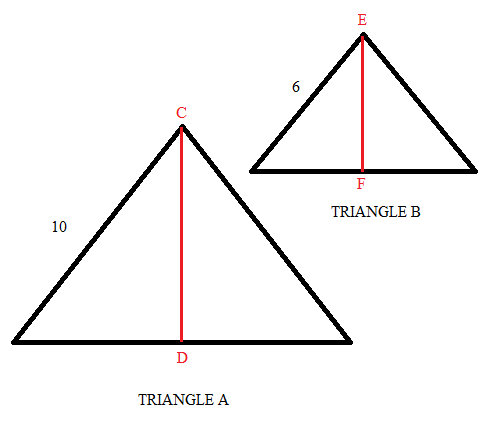#### Question 9 9. The two triangles shown are similar. The green lines represent the angle bisectors. What is the length of side x?### Page 3

#### Question 11 11. In the similar rectangles pictured below, what is y?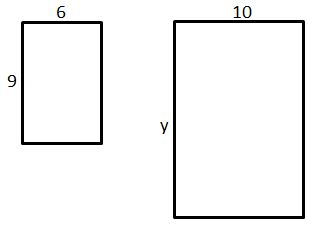#### Question 12 12. Which answer choice is similar to the triangle pictured below?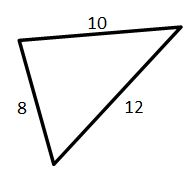#### Question 13 13. If triangle MNO is congruent to triangle PQR, then what is the measure of angle x?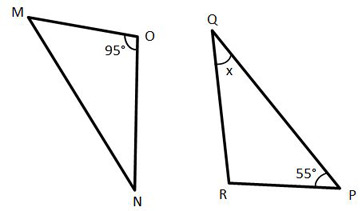#### Question 14 14. If the two triangles pictured below are similar, then what is x?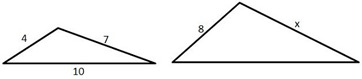#### Question 15 15. If the two triangles pictured below are congruent, then which angles are the same?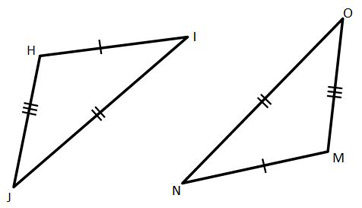### Page 4

#### Question 18 18. What shape do you see in this tessellation?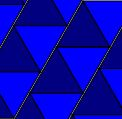### Page 5

#### Question 22 22. Which of the following is similar to this one?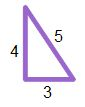#### Question 25 25. Which of these shapes is similar to this one?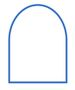### Page 6

#### Geometry of Congruent & Similar Figures Chapter Exam Instructions

Choose your answers to the questions and click 'Next' to see the next set of questions. You can skip questions if you would like and come back to them later with the yellow "Go To First Skipped Question" button. When you have completed the practice exam, a green submit button will appear. Click it to see your results. Good luck!

Support